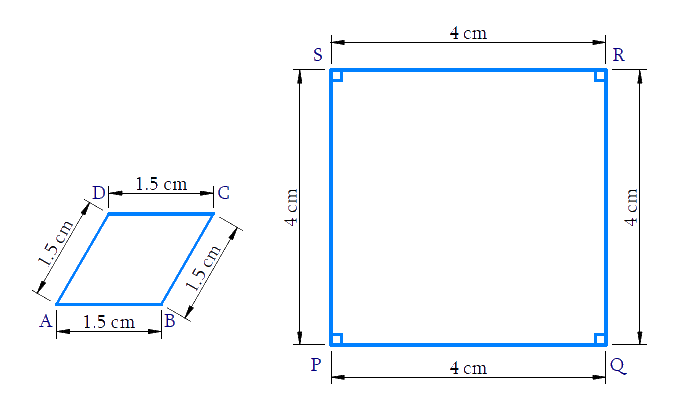# Ex.6.1 Q3 Triangles Solution - NCERT Maths Class 10

Go back to  'Ex.6.1'

## Question

State whether the following quadrilaterals are similar or not:Video Solution
Triangles
Ex 6.1 | Question 3

## Text Solution

Reasoning:

Two polygons of the same number of sides are similar, if

(i) all the corresponding angles are equal and

(ii) all the corresponding sides are in the same ratio (or proportion).

Steps:

In Quadrilaterals $$ABCD$$ and $$PQRS$$

\begin{align}\frac{{AB}}{{PQ}} = \frac{{BC}}{{QR}} = \frac{{CD}}{{RS}} = \frac{{DA}}{{SP}} = \frac{{3}}{{8}}\end{align}

$$\Rightarrow$$ Corresponding sides are in proportion

But $$\angle A \ne \angle P;\angle B \ne \angle Q$$

$$\Rightarrow$$ Corresponding angles are not equal

$$\square ABCD \nsim \square PQRS$$

No, Quadrilateral $$ABCD$$ is not similar to Quadrilateral $$PQRS$$

Learn from the best math teachers and top your exams

• Live one on one classroom and doubt clearing
• Practice worksheets in and after class for conceptual clarity
• Personalized curriculum to keep up with school